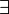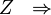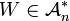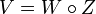# Notes for AKT-091020/0:10:00

Proof: 1)$\ \exists$ UFTI$Z \ \ \Rightarrow \$ fundamental theorem
Given$W \in \mathcal{A}_n^*$, set$V = W \circ Z$